# [KOF Arena] The high-level combo technique of cool guy ‘Kusanagi Kyo’

## Connecting the ‘Unguardable combo’ that can’t be avoided with emergency dodge

As the connection speed of Kyo isn’t that fast, the opponent can react quickly after seeing Kyo’s motion, so if you learn irregular combos when the opponent has emergency dodge and escape, you can pressure your opponent with force to gain the upper hand.

 Dokugami (W1) → Aragami (W2) → Kotosuki You (E1) → R.E.D. Kick (E2) → Aragami (A1) → Yamibarai (Q)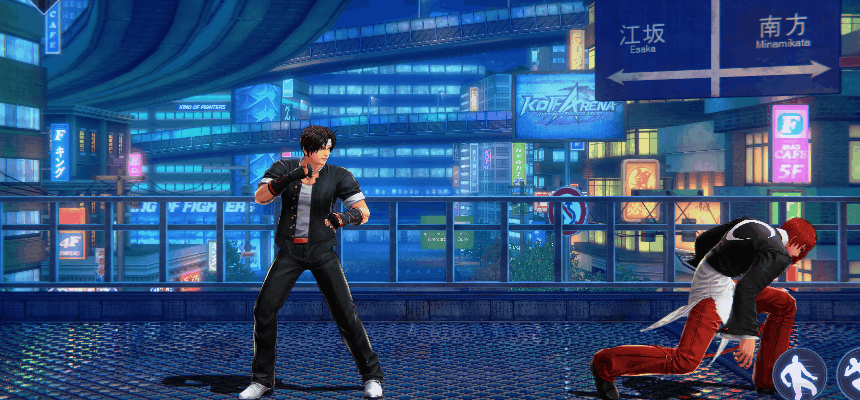▲ If use this combo when the opponent doesn’t have escape and only has emergency dodge, they can’t do anything.

## 'Mixing 1 basic attack' technique

The 4th attack Oniyaki of the basic attack (A), the 4th attack Nanase of Dokugami (W), and the R.E.D. Kick that follows Kotosuki You (E) have an airborne effect. To Kyo, making the opponent airborne could cause a problem since it gives space for the opponent to dodge. To connect combos smoothly, you need to force the airborne opponent into a standing state with Dokugami (W). Remember one more thing after this.

"If the opponent is airborne, use ‘1 basic attack (A1, Aragami)’ before connecting Dokugami (W).”

 Kotosuki You (E1) → R.E.D. Kick (E2) → Aragami (A1) → Dokugami (W1) → Aragami (W2) → Konoziku (W3) → Nanase (W4)

## ‘Advanced Combo’ techniques that mix 1 basic attack

 Aragami (A1) → Yanosabi (A2) → Dokugami (W1) → Aragami (W2) → Konokizu (W3) → Nanase (W4) → Aragami (A1) → Kotosuki You (E1) → R.E.D. Kick (E2) → Aragami (A1) → Yamibarai (Q)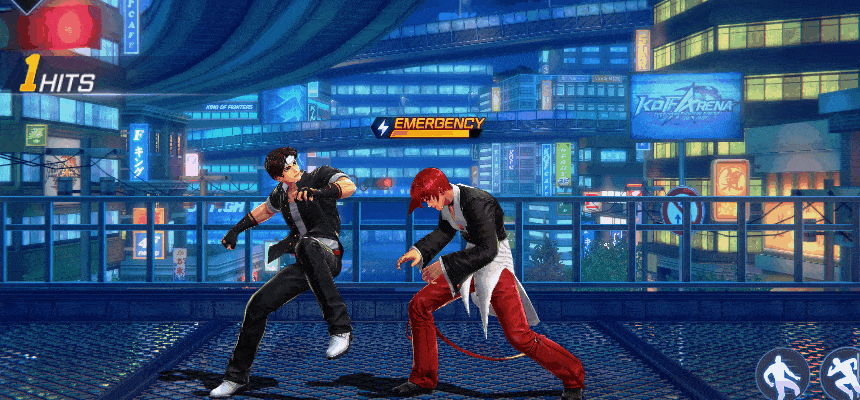▲ After the airborne effect of Nanase and R.E.D. Kick, mixing 1 basic attack (A1, Aragami) is key.

## Basic combo that begins with ‘Kotosuki You’

Connecting 3 basic attacks can be easily done by beginners as well. Try starting with skill attacks. If you get used to controlling Kyo, you should play irregular combos or mix basic attacks according to the situation.

 Kotosuki You (E1) → R.E.D. Kick (E2) → Dokugami (W1) → Aragami (W2) → Konokizu (W3) → Nanase (W4) → Yamibarai (Q)

## ‘Kotosuki You’ big hit combo that induces emergency dodge and escape

 Kotosuki You (E1) → R.E.D. Kick (E2) → Aragami (A1) → Dokugami (W1) → Aragami (W2) → Konokizu (W3) → Aragami (A1) → Yanosabi (A2) → 88 Shiki (A3) → Oniyaki (A4) → Aragami (A1) → Yamibarai (Q)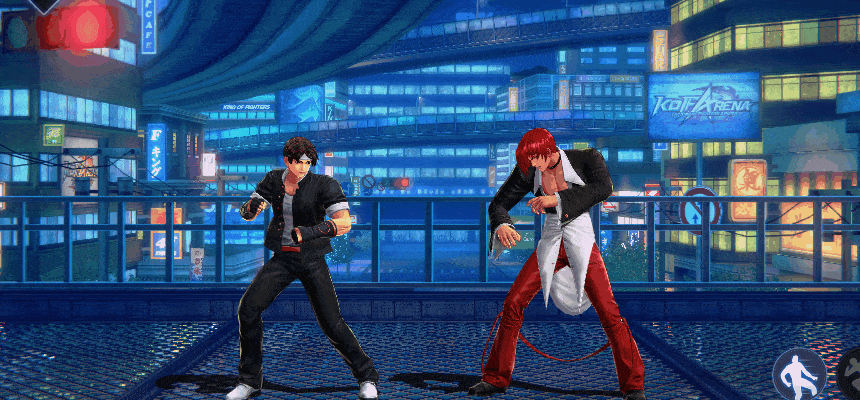▲ A combo that doesn’t cancel the 4th basic attack Oniyaki to induce emergency dodge or escape and continue pressuring.

## If the opponent doesn’t have emergency dodge? ‘Kotosuki You death combo’

 Kotosuki You (E1) → R.E.D. Kick (E2) → Aragami (A1) → Dokugami (W1) → Aragami (W2) → Konokizu (W3) → Aragami (A1) → Yanosabi (A2) → 88 Shiki (A3) → Oniyaki (A4, Cancel) → Yamibarai (Q) → Aragami (A1) → Yanosabi (A2) → 88 Shiki (A3) → Oniyaki (A4) → Aragami (A1) → Kotosuki You (E1) → R.E.D. Kick (E2) → Aragami (A1) → Dokugami (W1) → Aragami (W2) → Konokizu (W3)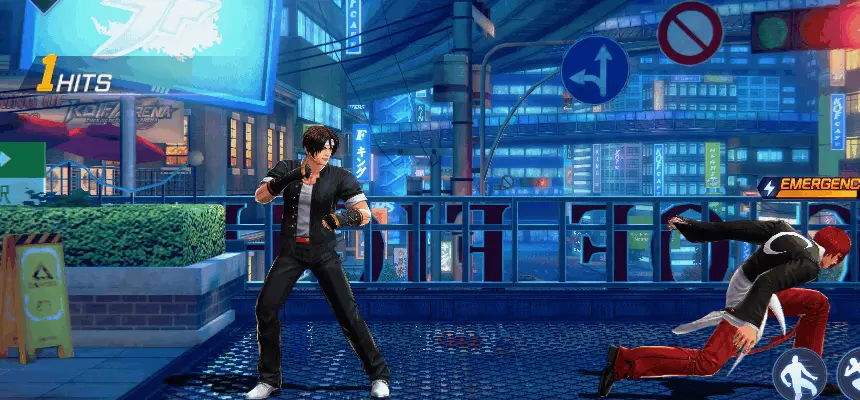▲ An infinite combo that keeps getting pounded if you don’t have emergency dodge and escape

## ‘Irregular combo’ to use when the opponent’s emergency dodge is at about half cooldown

You need to adjust the skill order according to the opponent’s ‘emergency’ gauge above their head. Especially, you need to control the cancel timing of Dokugami. This forces the opponent into a standing state, so if the combo gets long, you give them a gap where they can use dodge. Therefore, keep an eye on your opponent’s emergency gauge. When it’s almost refreshed, conclude the combo with a different skill.

 Aragami (A1) → Yanosabi (A2) → 88 Shiki (A3) → Oniyaki (A4) → Aragami (A1) → Dokugami (W1) → Aragami (W2) → Konokizu (W3) → Kotosuki You (E1) → R.E.D. Kick (E2) → Aragami (A1) → Yamibarai (Q)

## ‘Big hit combo’ to use right after the opponent uses emergency dodge

 Aragami (A1) → Yanosabi (A2) → 88 Shiki (A3) → Oniyaki (A4) → Aragami (A1) → Dokugami (W1) → Aragami (W2) → Konokizu (W3) → Aragami (A1) Kotosuki You (E1) → R.E.D. Kick (E2) → Aragami (A1) → Yamibarai (Q)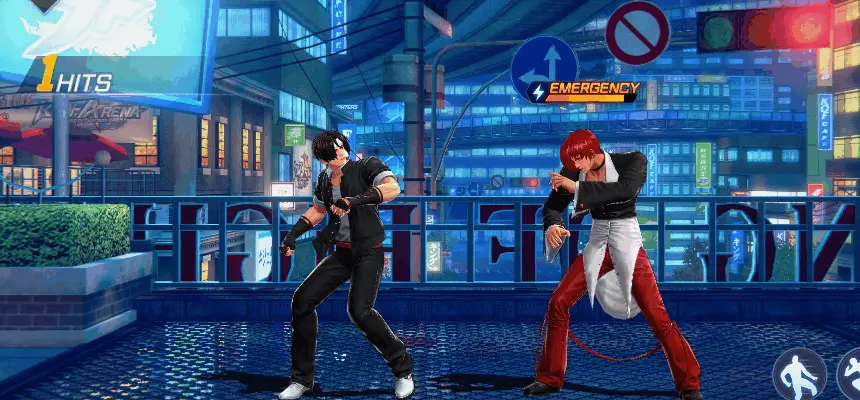▲ A big hit combo that doesn’t cancel the motion of the 4th basic attack Oniyaki
 Aragami (A1) → Yanosabi (A2) → 88 Shiki (A3) → Oniyaki (A4, Motion Cancel) → Yamibarai (Q) → Aragami (A1) → Yanosabi (A2) → 88 Shiki (A3) → Oniyaki (A4) → Aragami (A1) → Dokugami (W1) → Aragami (W2) → Konokizu (W3) → Aragami (A1) → Yanosabi (A2) → 88 Shiki (A3) → Oniyaki (A4) → Aragami (A1) → Kotosuki You (E1) → R.E.D. Kick (E2) → Aragami (A1) → Yamibarai (Q)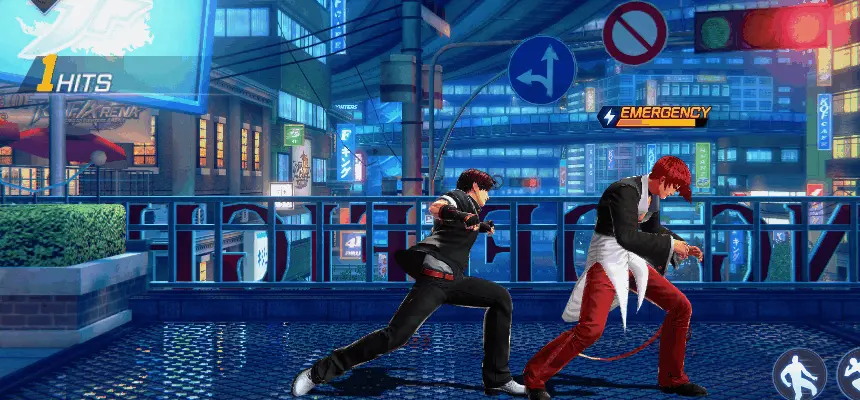▲ If you cancel the motion of the 4th strike Oniyaki, you can deal more damage.

## ‘Orochinagi big hit combo’ to use when the opponent doesn’t have escape

 Orochinagi (R) → Aragami (A1) → Dokugami (W1) → Aragami (W2) → Konokizu (W3) → Aragami (A1) → Yanosabi (A2) → 88 Shiki (A3) → Oniyaki (A4) → Aragami (A1) → Kotosuki You (E1) → R.E.D. Kick (E2) → Aragami (A1) → Yamibarai (Q)

## ‘Orochinagi death combo’ that sends your opponent to hell

 Orochinagi (R) → Aragami (A1) → Dokugami (W1) → Aragami (W2) → Konokizu (W3) → Aragami (A1) → Yanosabi (A2) → 88 Shiki (A3) → Oniyaki (A4, Motion Cancel) → Yamibarai (Q) → Aragami (A1) → Yanosabi (A2) → 88 Shiki (A3) → Oniyaki (A4) → Aragami (A1) → Kotosuki You (E1) → R.E.D. Kick (E2) → Aragami (A1) → Dokugami (W1) → Aragami (W2) → Orochinagi

•Sort by:

## Insert Image